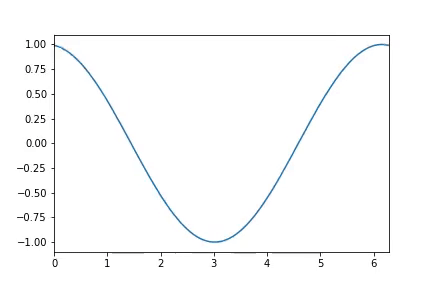Related Articles

# Create an Animated GIF Using Python Matplotlib

• Difficulty Level : Medium
• Last Updated : 14 Sep, 2021

In this article, we will discuss how to create an animated GIF using Matplotlib in Python.

Matplotlib can be used to create mathematics-based animations only. These can include a point moving on the circumference of the circle or a sine or cosine wave which are just like sound waves. In Matplotlib we have a library named animation from which we can import a function named as FuncAnimation(). This function is used to create animations. This function is used to call an animationFunction at a particular interval with a Frame number each time and displays the output of AnimationFunction in the Figure. Hence, this function mainly takes these four as its input.

Syntax:

` FuncAnimation( Figure, AnimationFunction, Frames, Interval)`

Also, there are other functions and objects too, which together make animation possible. They are given below:

1. We will require NumPy for various mathematical functions and arrays.
2. At last, we will require the plotting ability of matplotlib which is to be imported from its pyplot module.

Importing the required modules

```import numpy as np
from matplotlib.animation import FuncAnimation
from IPython import display
import matplotlib.pyplot as plt```

### Getting Started:

• The idea is to first create a simple plot of any function (here we had taken e.g. of Cosine ) and then FuncAnimation function will go on calling AnimationFunction passed to it as a parameter after a given interval continuously.
• We only have to give such an implementation that will result in a change in the position of the plot in our AnimationFunction which we had passed. And since the interval will be too small (in milliseconds) so we feel like it’s animating.

This is the basic idea for creating animations.

### Creating Animations:

We are going to create a cosine wave that is displayed in a video animation format.

The various steps and ideas used are listed below.

• Create a figure where the animation is to be displayed along with the x-axis and y-axis. This is done by creating a plot where we can put limits to x and y axes.

## Python3

 `Figure ``=` `plt.figure()` `# creating a plot``lines_plotted ``=` `plt.plot([])    ` `# putting limits on x axis since``# it is a trigonometry function``# (0,2∏)``line_plotted ``=` `lines_plotted[``0``]` `plt.xlim(``0``,``2``*``np.pi) ` `# putting limits on y since it is a``# cosine function``plt.ylim(``-``1.1``,``1.1``)   ` `# initialising x from 0 to 2∏``x ``=` `np.linspace(``0``,``2``*``np.pi,``100``)  ` `#initially``y ``=` `0`
• Now let’s create our AnimationFunction which will change the x and y coordinates of the plot continuously based on parameter frames. The reason is that FuncAnimation will call this function continuously according to frames. Since animation simply means that frames are put one after another to create a video.

## Python3

 `# function takes frame as an input``def` `AnimationFunction(frame):` `    ``# setting y according to frame``    ``# number and + x. It's logic``    ``y ``=` `np.cos(x``+``2``*``np.pi``*``frame``/``100``)` `    ``# line is set with new values of x and y``    ``line_plotted.set_data((x, y))`
• Now it’s time to call our FuncAnimation function which will call the above-defined function continuously as the number of frames. We are giving an interval of 25 milliseconds.

anim_created = FuncAnimation(Figure, AnimationFunction, frames=100, interval=25)

• Now it’s time to display our animation. So we have to make an HTML file out of it, through the below-given code:

## Python3

 `video ``=` `anim_created.to_html5_video()``html ``=` `display.HTML(video)``display.display(html)` `# good practice to close the plt object.``plt.close()`

Hence, the complete code can be run locally (if libraries are installed) or online on Jupyter Notebooks or Colaboratory Notebooks.

Output:Hence, we are able to create animation using Matplotlib which makes learning mathematics easy.

Attention geek! Strengthen your foundations with the Python Programming Foundation Course and learn the basics.

To begin with, your interview preparations Enhance your Data Structures concepts with the Python DS Course. And to begin with your Machine Learning Journey, join the Machine Learning – Basic Level Course

My Personal Notes arrow_drop_up